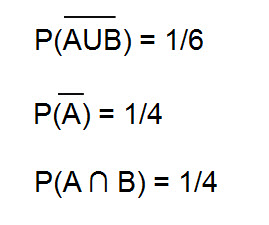Variables and events problem 1

Question (expected values problems): Below information is provided for events A and B.What could be inferred about A and B

a) A and B are independent and equally likely

b) A and B are independent and not equally likely

c) A and B are Mutually exclusive and equally likely

d) A and B are dependent and equally likely

Solution:

Calculate P(A U B) and P(A) as their complement values are givenP(A U B)      =     P(A) + P(B) – P(A ∩ B)

5/6                 =     3/4 +   P(B) –   1/4

P(B)              =       1/3

P(A) * P(B)  =     3/4 * 1/3 =   1/4

P(A ∩ B)       =      1/4 (Given)

P(A ∩ B) = P(A) * P(B)  – Which means A and B are independent events

Also, since the probability of A and B are different, they are NOT equally likely.

A and B are independent and NOT equally likely events

Expected values problem 2

Question (expected values problems): A company has three brands in its portfolio. Forecasts (for the next one year) for the revenues of each of these brands is given below along with the standard deviation of revenue.Calculate the overall expected revenue for the company along with the standard deviation.

Solution:

Let X1, X2, X3 be the revenues from three brands and Y be the overall revenue of the company.

Y = X1 + X2 + X3

Expected value:

Expected value of Y,  E[Y] = E[X1 + X2 + X3] = E[X1] + E[X2] + E[X3]

E[Y] = 45 + 10 + 78 = \$ 133 mn

Variance:

V[Y] = V[X1] + V[X2] + V[X3]

= 16 + 1 + 81 = 98

SD [Y] = SQRT(98) = \$ 9.9 mn

Hence, the overall expected revenue for the company over the next one year is \$ 133 mn with standard deviation of \$ 9.9 mn